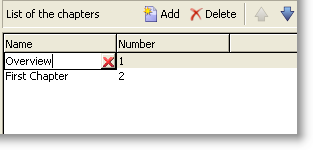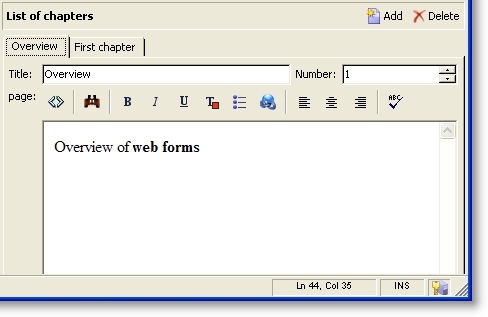v7 v8

# 输入表单

## 窗体结构

``````<form name="form_name" namespace="name_space">
…
</form>
````````````<form name="book" namespace="cus" type="contentForm">
<input xpath="@name"/>
<input xpath="@date"/>
<input xpath="@language"/>
</form>
``````

XPath是一种语法，允许您在XML文档的树中查找节点。

• @date：选择名为“date”的属性
• chapter/@title：选择“标题”属性位于 `<chapter>` 元素
• …/@date：从当前元素的父元素中选择日期

## 列表类型控件

### 列列表``````<input xpath="chapter" type="list">
<input xpath="@name"/>
<input xpath="@number"/>
</input>
````````````<input nolabel="true" toolbarCaption="List of chapters" type="list" xpath="chapter">
<input xpath="@name"/>
<input xpath="@number"/>
</input>
``````

toolbarCaption 属性强制水平对齐工具栏并填充列表上方的标题。

#### 放大列表

• 为了便于信息输入，
• 存在多线路控件，
• 列表中的列仅包含主字段，表单将显示收集要素的所有字段。``````<input nolabel="true" toolbarCaption="List of chapters" type="list" xpath="chapter" zoom="true" zoomOnAdd="true">
<input xpath="@name"/>
<input xpath="@number"/>

<form colcount="2" label="Editing a chapter">
<input xpath="@name"/>
<input xpath="@number"/>
<input colspan="2" xpath="page"/>
</form>
</input>
``````

A Detail 按钮添加时机 zoom="true" 属性在列表定义中输入。 这允许您在选定行上打开编辑表单。

### 选项卡列表``````<container toolbarCaption="List of chapters" type="notebooklist" xpath="chapter" xpath-label="@name">
<container colcount="2">
<input xpath="@name"/>
<input xpath="@number"/>
<input colspan="2" xpath="page"/>
</container>
</container>
``````

## 编辑窗体Preview 选项卡用于查看输入表单：